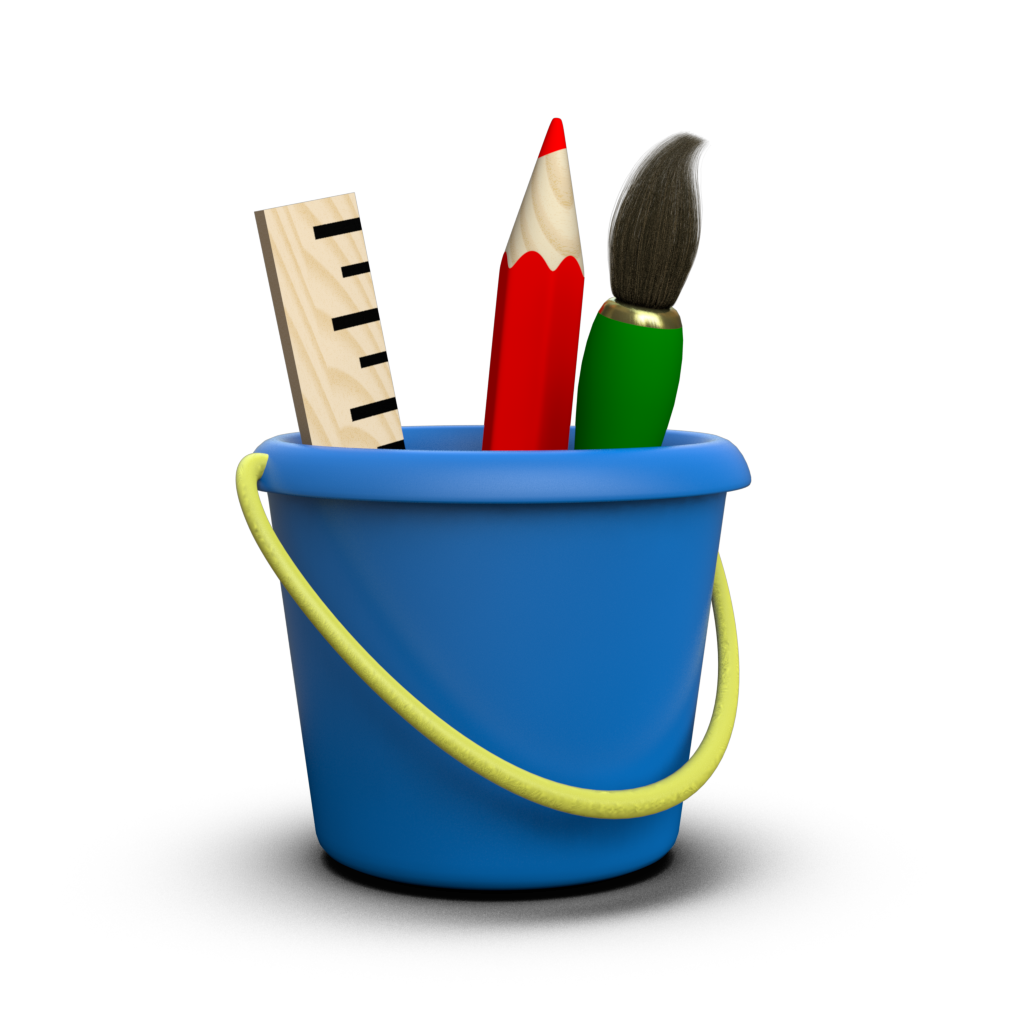# Convenience API¶

With Toyplot, a figure always consists of three parts:

Creating these entities separately gives you the maximum flexibility, allowing you to add multiple (even overlapping) coordinate systems to one canvas, splitting the marks among the different coordinate systems, etc. However for simple figures containing a single coordinate system and a single mark, this way of working can be tedious. Toyplot’s convenience API combines the three calls to create canvas, coordinate system, and mark into a single function that can handle many of your plotting needs with a minimum of code.

Consider the following verbose example:

:

import numpy
y = numpy.linspace(0, 1, 20) ** 2

:

import toyplot
canvas = toyplot.Canvas(width=300)
axes = canvas.cartesian()
axes.plot(y);


Using the convenience API, it can be reduced to a single call to toyplot.plot():

:

canvas, axes, mark = toyplot.plot(y, width=300)


Of course, if you’re using the convenience API there’s a good chance you don’t need the function’s return value (a (canvas, axes, mark) tuple) at all, making it even more compact:

:

toyplot.plot(y, width=300);


If you check the reference documentation for toyplot.plot(), you will see that its parameters include the union of the parameters for toyplot.canvas.Canvas, toyplot.canvas.Canvas.cartesian(), and toyplot.coordinates.Cartesian.plot(), except where parameter names might conflict.

Similar convenience API functions are provided for bar, fill, and scatter plots:

:

toyplot.bars(y, width=300);

:

toyplot.fill(numpy.column_stack((y, y*2)), width=300);

:

numpy.random.seed(1234)
toyplot.scatterplot(numpy.random.normal(size=50), width=300);

:

toyplot.matrix(numpy.random.normal(size=(10, 10)), width=300);

:

data = toyplot.data.cars()
columns = ["Year", "MPG", "Model"]
canvas, table = toyplot.table(data[:10, columns], width=300)
table.cells.column.width = 130


If you need greater control over the positioning of the axes within the canvas, need to add multiple axes to one canvas, or need to add multiple marks to one set of axes, you’ll have to create the canvas and axes explicitly.